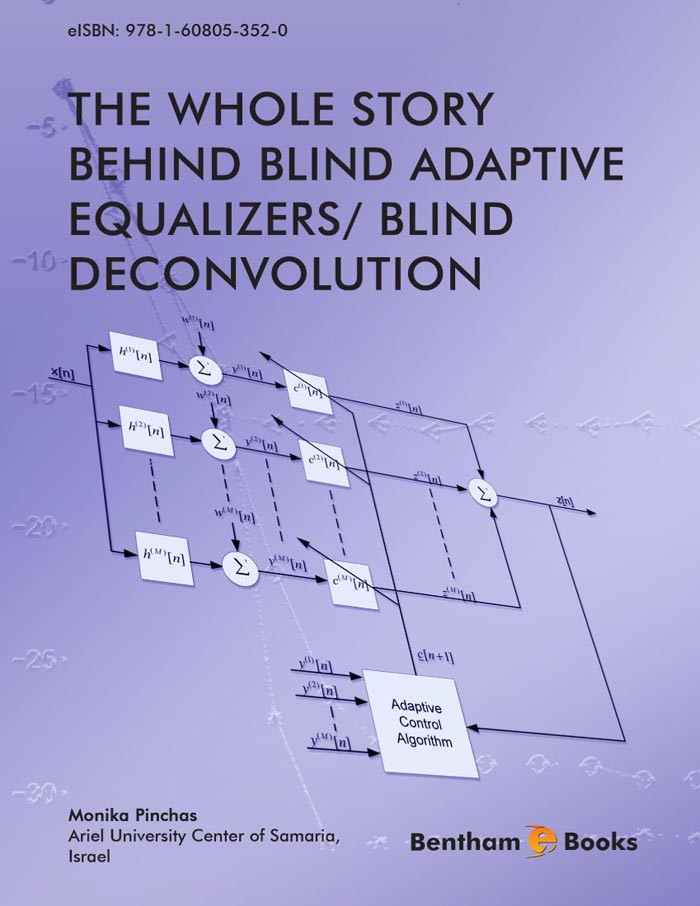Editor: Monika Pinchas

### The Whole Story Behind Blind Adaptive Equalizers/ Blind Deconvolution

eBook: US \$34 Special Offer (PDF + Printed Copy): US \$136
Printed Copy: US \$119
ISBN: 978-1-60805-135-9 (Print)
ISBN: 978-1-60805-352-0 (Online)
Year of Publication: 2012
DOI: 10.2174/97816080535201120101

#### Introduction

It is well known that Intersymbol (ISI) Interference is a limiting factor in many communication environments where it causes an irreducible degradation of the bit error rate (BER) thus imposing an upper limit on the data symbol rate. In order to overcome the ISI problem, an equalizer is implemented in those systems. Among the three types of equalizers - non-blind, semi-blind and blind – the blind equalizer has the benefit of bandwidth saving and there is no need of going through a training phase. Blind equalization algorithms are essentially adaptive filtering algorithms designed such that they do not require the external supply of a desired response to generate the error signal in the output of the adaptive equalization filter. The algorithms generate an estimate of the desired response by applying a nonlinear transformation to sequences involved in the adaptation process. This nonlinearity is designed to minimize a cost function that is implicitly based on higher order statistics (HOS) according to one approach, or calculated directly according to the Bayes rules.

The Whole Story behind Blind Adaptive Equalizers/ Blind Deconvolution gives the readers a full understanding on blind deconvolution. The e-book covers a variety of blind deconvolution/equalization methods based on both cost functions and Bayes rules where simulation results are supplied to support the theory. These include the Maximum Entropy density approximation technique and the Edgeworth Expansion approach used in various blind equalizers. It also describes the relationship between the cost function approach and the approach taken according to Bayes rules. The e-book deals also with the effect of various system parameters (such as the step-size parameter or the equalizer's tap length) have on the obtained equalization performance. This e-book will be of particular interest to advanced communications engineering undergraduate students, graduate students, university instructors and signal processing researchers.

Indexed in: Scopus, EBSCO.

# Preface

The problem of blind deconvolution arises comprehensively in various applications such as digital communications, seismic signal processing, speech modeling and synthesis, ultrasonic nondestructive evaluation and image restoration. Many papers are written on blind adaptive equalizers using the cost function approach or Bayes rules. But, up to now there is no book available that makes order in the various techniques, namely, explains the differences, advantages, disadvantages and relationship between the cost function and Bayesian approach.

It is well known that the equalizer's tap length, step-size parameter, channel power and source signal have a great affect on the convergence speed as well as on the residual Intersymbol Interference (ISI). Thus, it is important to understand the relationship between the equalization performance and the various parameters involved in the system in order to obtain optimal equalization performance from the residual ISI point of view and convergence speed. In this book, a whole chapter deals with the relationship between the equalizer's parameters and the equalization performance. Closed-form approximated expressions are given for the mean square error (MSE) as a function of time (for the real valued and two independent quadrature carrier case and low ISI) and residual ISI for type of serially blind adaptive equalizers where the error that is fed into the adaptive mechanism which updates the equalizer's taps can be expressed as a polynomial function of order three of the equalized output.

The book explains why higher order statistics (HOS) are used in the blind equalization eld and why HOS based blind equalization methods can not be applied to Gaussian sources. In addition, it explains the two di erent classes of HOS-based equalization methods used in the literature and supplies examples for each class.

The book describes the single input single output (SISO) and single input multiple output (SIMO) system where the condition for perfect equalization performance from the residual ISI point of view is given for each case.

In the literature, the convolutional noise is often assumed to be a Gaussian process. In this book we propose a new model for the convolutional noise probability density function (pdf). The new model is based on the Edgeworth expansion which is close related to the Gaussian model. Based on this new model for the convolutional noise pdf, a new closed-form approximated expression for the conditional expectation is obtained. It should be pointed out that the new derived expression for the conditional expectation, is also based on the Maximum Entropy approach, Laplace integral method and is valid for the noiseless, real valued and two independent quadrature carrier case. According to simulation results carried out for signal to noise ratio (SNR) SNR = 30 [dB], the new derived expression leads to improved equalization performance for the 16QAM input constellation case.

In summary, this book not only explains the whole story behind blind adaptive equalizers/blind deconvolution in such a way, that also a beginner in this eld will feel comfortable with the book but also supplies new developments, new ideas where simulation results are supplied to support the theory and a simple Matlab program for a blind adaptive equalizer (Godard's algorithm) for the 16QAM constellation input which is sent via an easy channel (FIR channel). This book can be used as a teaching book and may be of particular interest to advanced undergraduate students, graduate students, university instructors and research scientists in related disciplines.

MONIKA PINCHAS
Department of Electrical and Electronic Engineering, Ariel University
Center of Samaria, Ariel 40700, ISRAEL
monika.pinchas@gmail.com